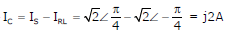# Electric Circuits - Online Test

Q1. The r.m.s value of the current i(t) in the circuit shown below is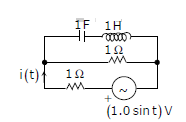Explaination / Solution: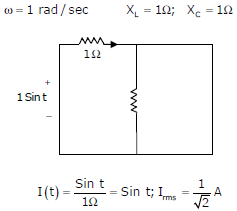Q2. The voltage applied to a circuit is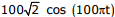volts and the circuit draws a current ofamperes. Taking the voltage as the reference phasor, the phasor representation of the current in amperes is
Explaination / Solution: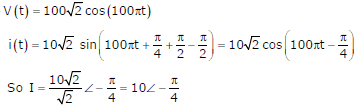Q3.
In the circuit given below, the value of R required for the transfer of maximum power to the load having a resistance of 3W is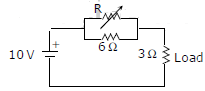Explaination / Solution: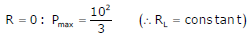Q4. A lossy capacitor Cx, rated for operation at 5 kV, 50 Hz is represented by an equivalent circuit with an ideal capacitor Cp in parallel with a resistor Rp. The value Cp is found to be 0.102 µF and the value of Rp = 1.25 MΩ . Then the power loss and tan ∂ of the lossy capacitor operating at the rated voltage, respectively,
Explaination / Solution:
No Explaination.

Q5. A capacitor is made with a polymeric dielectric having an εr of 2.26 and a dielectric breakdown strength of 50kV/cm. The permittivity of free space is 8.85pF/m. If the rectangular plates of the capacitor have a width of 20cm and a length of 40cm, then the maximum electric charge in the capacitor is
Explaination / Solution: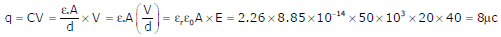Q6.
A three – bus network is shown in the figure below indicating the p.u. impedances of each element.The bus admittance matrix, Y – bus, of the network is
Explaination / Solution:Q7. The input voltage given to a converter is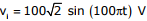The current drawn by the converter isThe input power factor of the converter is
Explaination / Solution: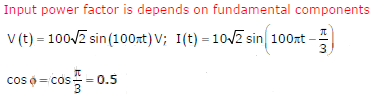Q8. The input voltage given to a converter isThe current drawn by the converter isThe active power drawn by the converter is
Explaination / Solution:Q9. An RLC circuit with relevant data is given below.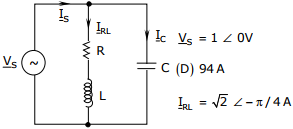The power dissipated in the resistor R is
Explaination / Solution: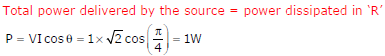Q10. An RLC circuit with relevant data is given below.The current Ic in the figure above is
Explaination / Solution: